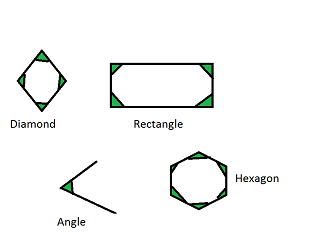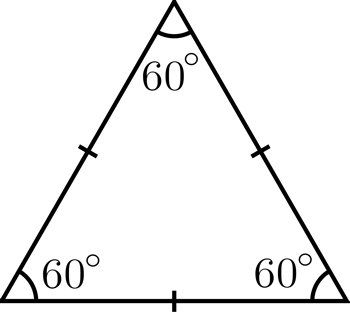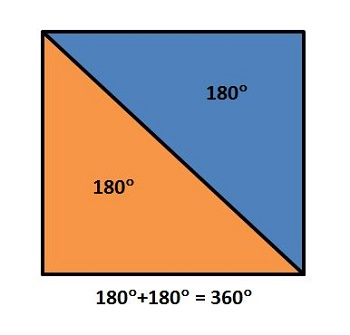# How to Find Interior Angles

Instructor: Nola Bridgens

Nola has taught elementary school and tutored for four years. She has a bachelor's degree in Elementary Education, a master's degree in Marketing, and is a certified teacher.

After completing this lesson, you will be able to describe what an interior angle is and find its measurements. We will explore interior angles in regular polygons such as equilateral triangles, squares, and pentagons.

## What is an Interior Angle?

Interior angles are angles inside of a shape. We find interior angles in triangles, quadrilaterals, and any other type of polygon. Interior angles can be acute like the angles in a diamond, obtuse like the angles in a hexagon, or right angles like those in a rectangle.## What is a Regular Polygon?

A regular polygon is a shape in which all sides are equal and all angles are equal. A square is an example of a regular polygon because all sides of a square are the same length and all the angles are 90o. The hexagon in the diagram above is a regular polygon because all the sides and all the angles are equal.

## Interior Angles of an Equilateral Triangle

The sum of the angles in a triangle always equal 180o. In a regular (or equilateral) triangle, all the sides and all the angles are equal. When all the angles are the same, we can divide the sum of the angles by the number of sides. The sum of the angles in a triangle is 180o and it has 3 sides: 180o/ 3 = 60o. All the angles in an equilateral triangle are 60o.## Interior Angles of a Square

Squares have 4 equal sides and the sum of the angles always equals 360o. This is easy to picture when you cut a square in half diagonally--it's made up of two triangles. If a triangle has an angle sum of 180o, it makes sense that, because there are two triangles in a square, the sum of the angles in a square is 360o (180o +180o).

To find the measurement of the interior angles in a square, you divide the sum of the angles (360o) by the number of sides (4). So, 360o / 4 = 90o. All the angles in a square are equal to 90o.## Interior Angles of Other Polygons

The general rule is that every time you add another side to a shape, the sum of the angles increases by 180o. For example when you go from a 3-sided triangle (180o) to a 4-sided square, you add 180o to the sum of the angles. Therefore, a square has angles with a sum of 360o.

To unlock this lesson you must be a Study.com Member.

### Register to view this lesson

Are you a student or a teacher?

#### See for yourself why 30 million people use Study.com

##### Become a Study.com member and start learning now.
Back
What teachers are saying about Study.com

### Earning College Credit

Did you know… We have over 200 college courses that prepare you to earn credit by exam that is accepted by over 1,500 colleges and universities. You can test out of the first two years of college and save thousands off your degree. Anyone can earn credit-by-exam regardless of age or education level.